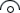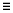# Extract Points and Nodes

Use the Extract Points/Nodes tool to interpolate and divide an edge or line, and find the center of an arc, fillet, or multiple curve segments.

1. From the Geometry ribbon, click the arrow next to the Create tool set, then select Extract Points.
2. From the drop-down menu below the tool, select whether to work with points or nodes.
3. Extract points/nodes in the following ways:
Option Description
Interpolate Points/Nodes
1. Click on a line.
2. In the microdialog, enter the number of points/nodes to extract and press Enter.

New points are organized in the same component as their source line.

Tip:
• Re-interpolate the number of points/nodes on the selected line by entering a new value in the microdialog and pressing Enter.
• When interpolating from multiple lines, the same number of points/nodes will be created on each line and distributed evenly along their respective source line’s length.
• When interpolating from a closed loop, the start and end points/nodes are the same, but separate points/nodes will be created at each location. To divide a circle into four equal lines, interpolate five points/nodes.
Find Curve Center
1. Click on an arc.
2. In the microdialog, click.
Note: When calculating the curve center of multiple lines, a single point/node is created at the best matching curve center of the combined lines.
Extract Parametric Create nodes at parametric locations on lines and surfaces.
1. From the guide bar, clickto define the U and V lower and upper bounds.
For lines
• 0.0 <= u lower bound <= u upper bound <= 1.0.
• If the lower and upper bounds are the same, only one node is created.
• If the number of u nodes is specified as 1, only the lower bound is used.
• The line parameterization type can be specified as either arc length or internal. Internal parameterizations depend on how the line was originally created, while arc length simply distributes the nodes uniformly.
For surfaces
• 0.0 <= u lower bound <= u upper bound <= 1.0.
• 0.0 <= v lower bound <= v upper bound <= 1.0.
• The number of u and v nodes must be >= 1.
• The total number of nodes created equals (number of u nodes * number of v nodes).
• The surface parametric area is scaled to the visible surface area. Nodes will be created inside the visible surface area.